§ 2   Singular integral equations

1. Definition and Examples of Singular Integral Equations

1 ° If the integral of the integral equation is a generalized integral with an infinite integral interval (or the kernel K ( x, ξ ) is an unbounded function), then the equation is called a singular integral equation, for example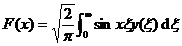(1)(2)

and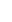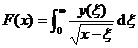(3)

All are singular integral equations.

The function defined on the right side of the equation ( 1 ) can be viewed as the Fourier sine transform of y(x) . If F(x) is segment-wise differentiable and exists when x > 0 , then equation ( 1 ) has a unique inversion formula: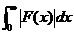( x > 0 )

Consider the homogeneous integral equation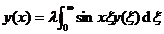(4)

from the known formula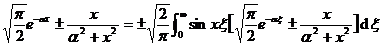( x > 0, α > 0 )

It can be seen that it is indeed an eigenvalue. At that time , for any constant α , the function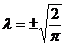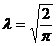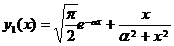( x > 0)

Equation ( 4 ) is satisfied; and at that time , for any constant α, the function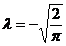( x > 0)

Equation ( 4 ) is also satisfied. Therefore, these two λ values ​​are infinitely heavy eigenvalues, that is, each value corresponds to an infinite number of eigenfunctions. This fact is quite different from the fact that any eigenvalue of the Fr equation corresponds to only a finite number of independent eigenfunctions.

3° The function   F ( x ) defined by the right-hand side of equation ( 2 ) is the Laplace transform of the function y ( x ). Because not all functions can be Laplace transformed, two different functions cannot have the same Laplace transform. So for a given function F(x) , if there is a solution to ( 2 ), the solution is unique.

Consider the homogeneous integral equation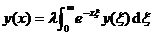( x > 0 ) ( 5 )

According to the definition of the gamma function, we have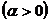to replace a , we get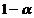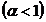Deduced from the above two equations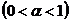if orderThen the above formula shows that the function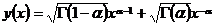( x > 0 )

is the solution of the integral equation ( 5 ).

Therefore, for any value of parameter a , there is a corresponding value of λ , which determines a non-trivial solution of equation ( 5 ).use identities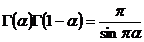have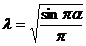It is deduced from this that all λ values ​​in the interval are the eigenvalues ​​of the singular integral equation ( 5 ).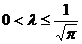It can also be shown that all λ values ​​in the interval are also eigenvalues ​​of the singular integral equation ( 5 ).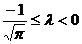The integral interval of integral equation ( 3 ) is finite, but the kernel is an unbounded function, and this singular integral equation will be considered in this section II and §3 .

2. Integral equations with Cauchy and Hilbert kernels

[ Cauchy core and Hilbert core ]

Theorem Let L be any smooth closed curve, a function defined on L that satisfies the Lipschitz condition * with exponent a , when z tends to any point t on L from the interior of L , then the Cauchy-type integral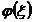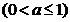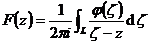( 1 )

approaching the limit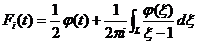As z tends to any point t on L from the outside of L , the integral ( 1 ) tends to the corresponding limit value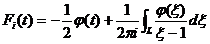The integrals in the above two equations are both generalized integrals

expression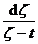Called the Cauchy kernel, where ζ and t are any two points on L.

expression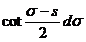It is called the Hilbert kernel, where both s and σ are real variables and vary within a closed interval .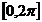There is a very simple relationship between the Cauchy kernel and the Hilbert kernel. Let L be a simple closed curve, which is a smooth curve with continuous curvature. Let the parametric equation of L be,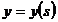Let the corresponding parameter s change in a closed interval . Let t=x + i y and t=x (s)+ i y (s) . The equation for L can be written as t=t ( s ) . Let ζ be any point on L , then there is a parameter value σ , so that . So it is not difficult to prove that: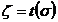where P ( s , σ ) is a continuous function of two variables s and σ , which satisfies the Lipschitz condition with a certain exponent.

[ Singular integral equation with Hilbert kernel ] Consider an equation of the form :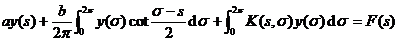(2)

where a and b are constants.

It is assumed that both the kernel K ( s , σ ) and the free term F ( s ) satisfy the Lipschitz condition.

If K ( s , σ ) 0 , then the equation under consideration is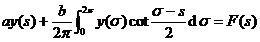(3)

If a 2 +b 20, the solution to this equation is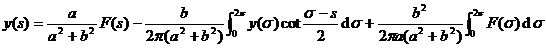(4)

If a 2 +b 2 =0, the equation generally has no solution.

Note the special case a = 0. If b = 1 is not guarded , then ( 3 ) becomes the first type of Fr equation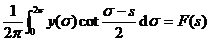At this time, the solution of equation ( 4 ) is useless, but the necessary and sufficient conditions for equation ( 3 ) to have a solution are :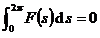The solution is of the formwhere C is an arbitrary constant.

In the general case, equation ( 2 ) can be shown to be equivalent to the general form of the Fr equation, and the equation under consideration then boils down to solving the Fr equation.

The general form of a singular integral equation with a Hilbert kernel is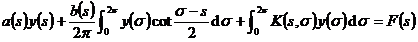where a ( s ) and b ( s ) are functions of variable s . If a ( s ) and b ( s ) satisfy the Lipschitz condition, the above equation can be reduced to the Fr equation, but the two may not be equivalent.

[ Singular integral equation with Cauchy kernel ]   Consider an equation of the form:( 5 )

where a and b are constants and L is a closed curve.

If a 2 - b 20, the solution of ( 5 ) is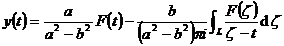The general form of a singular integral equation with a Cauchy kernel is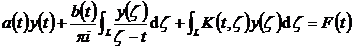It can also be reduced to the Fr equation. If a and b are constants, the resulting Fr equation is equivalent to the above equation. In general, additional explanations are required.

* If there are two constants K and a ( 0 < a1 ) , make the following inequality hold for any pair of values ​​on the interval [ a , b ] :

Then the function f ( x ) is said to satisfy the Lipschitz condition with exponent α .